# 采用栈式 RBM 的深度神经网络。自训练, 自控制

18 十月 2016, 10:35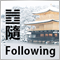9
25 526

### 目录

• 1. DBN 的结构
• 2. 数据的准备及选择
• 2.1. 输入变量
• 2.2. 输出变量
• 2.3. 初始化数据帧
• 2.3.1. 删除高相关性变量
• 2.4. 最重要变量的选择
• 3. 实验部分。
• 3.1. 构建模型
• 3.1.1. "darch" 软件包的简述
• 3.1.2. 构建 DBN 模型。参数。
• 3.2. 训练和测试样品的形成。
• 3.2.1. 分级平衡和预处理。
• 3.2.2. 目标变量编码
• 3.3. 训练模型
• 3.3.1. 预训练
• 3.3.2. 微调
• 3.4. 测试模型。标尺。
• 3.4.1. 预测解码。
• 3.4.2. 改善预测结果
• 校验
• 使用马尔科夫链模型进行平滑
• 在理论性平衡曲线上校正预测信号
• 3.4.3. 标尺
• 4. 智能交易程序的结构
• 4.1. 智能交易程序操作的描述
• 4.2. 自控制。自训练
• 安装和启动
• 改进定性指标的方式方法。
• 结论

### 1. 由栈式 RBM (DN_SRBM) 初始化的深层神经网络的结构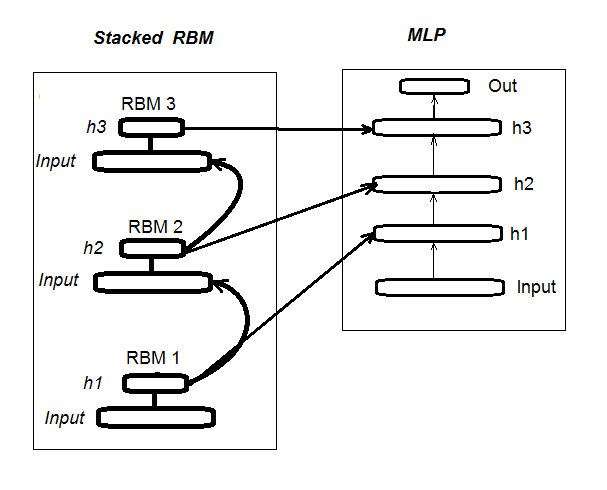### 2.1. 输入变量 (征兆, 预测器)

```#---2---------------------------------------------
In <- function(p = 16){
require(TTR)
require(dplyr)
require(magrittr)
adx <- ADX(price, n = p) %>% as.data.frame %>%
mutate(.,oscDX = DIp - DIn) %>%
transmute(.,DX, ADX, oscDX) %>%
as.matrix()
ar <- aroon(price[ ,c('High', 'Low')], n = p) %>%
extract(,3)
atr <- ATR(price, n = p, maType = "EMA") %>%
extract(,1:2)
cci <- CCI(price[ ,2:4], n = p)
chv <- chaikinVolatility(price[ ,2:4], n = p)
cmo <- CMO(price[ ,'Med'], n = p)
macd <- MACD(price[ ,'Med'], 12, 26, 9) %>%
as.data.frame() %>%
mutate(., vsig = signal %>%
diff %>% c(NA,.) %>% multiply_by(10)) %>%
transmute(., sign = signal, vsig) %>%
as.matrix()
rsi <- RSI(price[ ,'Med'], n = p)
stoh <- stoch(price[ ,2:4], nFastK = p,
nFastD =3, nSlowD = 3,
maType = "EMA") %>%
as.data.frame() %>%
mutate(., oscK = fastK - fastD) %>%
transmute(.,slowD, oscK) %>%
as.matrix()
smi <- SMI(price[ ,2:4],n = p, nFast = 2,
nSlow = 25, nSig = 9)
kst <- KST(price[ ,4])%>% as.data.frame() %>%
mutate(., oscKST = kst - signal) %>%
select(.,oscKST) %>% as.matrix()
In <- cbind(adx, ar, atr, cci, chv, cmo, macd,
rsi, stoh, smi, kst)
return(In)
}
```

### 2.2输出数据 (目标白能量)

```#----3------------------------------------------------
ZZ <- function(pr = price, ch = ch , mode="m") {
require(TTR)
require(magrittr)
if (ch > 1) ch <- ch/(10 ^ (Dig - 1))
if (mode == "m") {pr <- pr[ ,'Med']}
if (mode == "hl") {pr <- pr[ ,c("High", "Low")]}
if (mode == "cl") {pr <- pr[ ,c("Close")]}
zz <- ZigZag(pr, change = ch, percent = F,
retrace = F, lastExtreme = T)
n <- 1:length(zz)
dz <- zz %>% diff %>% c(., NA)
sig <- sign(dz)
for (i in n) { if (is.na(zz[i])) zz[i] = zz[i - 1]}
return(cbind(zz, sig))
}
```

pr = 价格 – OHLCMed 报价的矩阵;

ch – 之字折线弯曲的最小长度点数 (4 位) 或是实际项 (例如, ch = 0.0035);

mode – 应用的价格 ("m" - 中间价, "hl" - 最高价和最低价, "cl" - 收盘价), 省缺使用中间价。

```> out <- ZZ(ch = 37, mode = "m")
> table(out[ ,2])

-1    1
2828 3162
```

### 2.3. 初始化数据帧

```#-----4---------------------------------
form.data <- function(n = 16, z = 37, len = 500){
require(magrittr)
x <- In(p = n)
out <- ZZ(ch = z, mode = "m")
data <- cbind(x, y = out[ ,2]) %>%
as.data.frame %>% head(., (nrow(x)-len))%>%
na.omit
data\$y <- as.factor(data\$y)
return(data)
}
```

### 2.3.1. 删除高相关性变量

```> data <- form.data(n = 16, z = 37) # 准备数据帧
> descCor <- cor(data[ ,-ncol(data)])# 删除目标变量
> summary(descCor[upper.tri(descCor)])
Min. 1st Qu.  Median    Mean 3rd Qu.    Max.
-0.1887  0.0532  0.2077  0.3040  0.5716  0.9588
> highCor <- caret::findCorrelation(descCor, cutoff = 0.9)
> highCor
 12  9 15
> colnames(data[ ,highCor])
 "rsi" "cmo" "SMI"
```

```> data.f <- data[ ,-highCor]
> colnames(data.f)
 "DX"     "ADX"    "oscDX"  "ar"     "tr"
 "atr"    "cci"    "chv"    "sign"   "vsig"
 "slowD"  "oscK"   "signal" "vol"    "Class"
```

```#---5-----------------------------------------------
cleaning <- function(n = 16, z = 37, cut = 0.9){
data <- form.data(n, z)
descCor <- cor(data[ ,-ncol(data)])
highCor <- caret::findCorrelation(descCor, cutoff = cut)
data.f <- data[ ,-highCor]
return(data.f)
}
> data.f <- cleaning()
```

### 2.4. 最重要变量的选择

• 具有最佳贡献和交互的变量;
• 对于分级 "-1" 的最佳变量;
• 对于分级 "+1" 的最佳变量。
```#-----6------------------------------------------------
prepareBest <- function(n, z, cut, method){
require(randomUniformForest)
require(magrittr)
data.f <<- cleaning(n = n, z = z, cut = cut)
idx <- rminer::holdout(y = data.f\$Class)
prep <- caret::preProcess(x = data.f[idx\$tr, -ncol(data.f)], method = method)
x.train <- predict(prep, data.f[idx\$tr, -ncol(data.f)])
x.test <- predict(prep, data.f[idx\$ts, -ncol(data.f)])
y.train <- data.f[idx\$tr, ncol(data.f)]
y.test <- data.f[idx\$ts, ncol(data.f)]
#---------
ruf <- randomUniformForest( X = x.train, Y = y.train,
xtest = x.test, ytest = y.test,
mtry = 1, ntree = 300,
threads = 2, nodesize = 1
)
imp.ruf <- importance(ruf, Xtest = x.test)
best <- imp.ruf\$localVariableImportance\$classVariableImportance %>%
head(., 10) %>% rownames()
#-----部分重要
best.sell <- partialImportance(X = x.test,
imp.ruf,
whichClass = "-1",
nLocalFeatures = 7) %>%
row.names() %>%
as.numeric() %>%
colnames(x.test)[.]
best.buy <- partialImportance(X = x.test,
imp.ruf,
whichClass = "1",
nLocalFeatures = 7) %>%
row.names() %>%
as.numeric() %>%
colnames(x.test)[.]
dt <- list(best = best, buy = best.buy, sell = best.sell)
return(dt)
}
```

n – 输入数据参数;

z – 输出数据参数;

cut – 变量的相关性阀值;

method – 输入数据预处理方法。

• 创建初始的 data.f 集合, 高相关性变量已移除, 并将之保存以备后用;
• 识别训练索引和 idx 的测试样本;
• 判断 prep 的预处理参数;
• 将初始样本划分为训练和测试样本, 输入数据规范化;
• 在所获取的集合之上获取并测试 ruf 模型;
• 计算 imp.ruf 变量的重要性;
• 选择 10 个贡献和交互度最重要的变量 — 最佳;
• 选择 7 个对于 "-1" 和 "+1" 分级最重要的变量 — 最佳买入, 最佳卖出;
• 创建三个预测器的集合列表 — 最佳, 最佳买入, 最佳卖出。

```> dt <- prepareBest(16, 37, 0.9, c("center", "scale","spatialSign"))

作为 替换。

1 - 全局变量重要性 (14 基于所获信息最重要) :

变量得分 class class.frequency 百分比
1        cci  4406    -1            0.51  100.00
2     signal  4344    -1            0.51   98.59
3        ADX  4337    -1            0.51   98.43
4       sign  4327    -1            0.51   98.21
5      slowD  4326    -1            0.51   98.18
6        chv  4296    -1            0.52   97.51
7       oscK  4294    -1            0.52   97.46
8        vol  4282    -1            0.51   97.19
9         ar  4271    -1            0.52   96.95
10       atr  4237    -1            0.51   96.16
11     oscDX  4200    -1            0.52   95.34
12        DX  4174    -1            0.51   94.73
13      vsig  4170    -1            0.52   94.65
14        tr  4075    -1            0.50   92.49
percent.importance
1                   7
2                   7
3                   7
4                   7
5                   7
6                   7
7                   7
8                   7
9                   7
10                  7
11                  7
12                  7
13                  7
14                  7

2 - 局部变量重要性

cci  slowD    atr     tr     DX
atr          0.1804 0.1546 0.1523 0.1147 0.1127
cci          0.1779 0.1521 0.1498 0.1122 0.1102
slowD        0.1633 0.1375 0.1352 0.0976 0.0956
DX           0.1578 0.1319 0.1297 0.0921 0.0901
vsig         0.1467 0.1209 0.1186 0.0810 0.0790
oscDX        0.1452 0.1194 0.1171 0.0795 0.0775
tr           0.1427 0.1168 0.1146 0.0770 0.0750
oscK         0.1381 0.1123 0.1101 0.0725 0.0705
sign         0.1361 0.1103 0.1081 0.0704 0.0685
signal       0.1326 0.1068 0.1045 0.0669 0.0650
avg1rstOrder 0.1452 0.1194 0.1171 0.0795 0.0775
vsig  oscDX   oscK signal     ar
atr          0.1111 0.1040 0.1015 0.0951 0.0897
cci          0.1085 0.1015 0.0990 0.0925 0.0872
slowD        0.0940 0.0869 0.0844 0.0780 0.0726
DX           0.0884 0.0814 0.0789 0.0724 0.0671
vsig         0.0774 0.0703 0.0678 0.0614 0.0560
oscDX        0.0759 0.0688 0.0663 0.0599 0.0545
tr           0.0733 0.0663 0.0638 0.0573 0.0520
oscK         0.0688 0.0618 0.0593 0.0528 0.0475
sign         0.0668 0.0598 0.0573 0.0508 0.0455
signal       0.0633 0.0563 0.0537 0.0473 0.0419
avg1rstOrder 0.0759 0.0688 0.0663 0.0599 0.0545
chv    vol   sign    ADX avg2ndOrder
atr          0.0850 0.0850 0.0847 0.0802      0.1108
cci          0.0824 0.0824 0.0822 0.0777      0.1083
slowD        0.0679 0.0679 0.0676 0.0631      0.0937
DX           0.0623 0.0623 0.0620 0.0576      0.0881
vsig         0.0513 0.0513 0.0510 0.0465      0.0771
oscDX        0.0497 0.0497 0.0495 0.0450      0.0756
tr           0.0472 0.0472 0.0470 0.0425      0.0731
oscK         0.0427 0.0427 0.0424 0.0379      0.0685
sign         0.0407 0.0407 0.0404 0.0359      0.0665
signal       0.0372 0.0372 0.0369 0.0324      0.0630
avg1rstOrder 0.0497 0.0497 0.0495 0.0450      0.0000

cci    atr  slowD     DX     tr   vsig  oscDX
0.1384 0.1284 0.1182 0.0796 0.0735 0.0727 0.0677
oscK signal   sign
0.0599 0.0509 0.0464

Class -1 Class 1
cci        0.17    0.23
slowD      0.20    0.09
atr        0.14    0.15
tr         0.04    0.12
oscK       0.08    0.03
vsig       0.06    0.08
oscDX      0.04    0.08
DX         0.07    0.08
signal     0.05    0.04
ar         0.04    0.02
```

1. 在全局重要性方面, 所有 14 个输入变量相等。
2. 按照整体贡献 (全局重要性) 和交互性 (局部重要性) 定义的 10 个最佳。
3. 每个分级的七个最佳部分重要性变量显示在以下图表。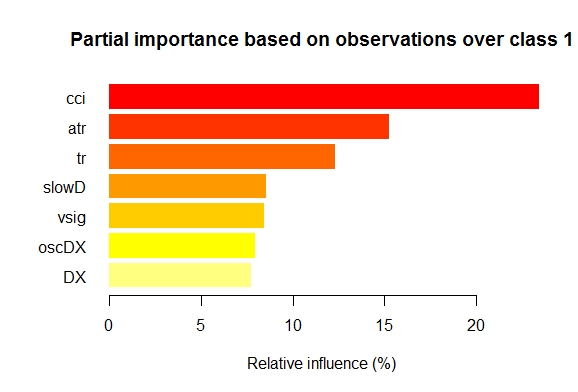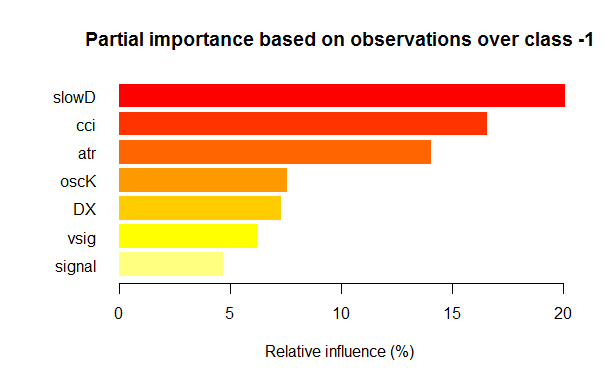### 3. 实验部分。

• 通过应用 Intel® 数学内核库 的多线程处理, 进行快速且更定性的计算;
• 可重现 R 工具箱高级功能。一个小小的澄清: R 语言正在积极发展, 不断改进现有软件包, 并添加新功能。这种进展的反面则涉及到可重现的丧失。也就是说, 您几个月之前编写的产品运行良好, 但在下次软件包更新后突然停工。大量的时间会浪费在识别并清除由更新的软件包导致的错误。例如, 第一篇深度神经网络文章附加的智能交易系统在创建之时功能良好。然而, 发布几个月之后一些用户抱怨它已不可运行。分析表明是更新的 "svSocket" 软件包引起智能交易系统的故障, 而且我无法找到其背后的原因。最后版本的智能交易系统将附加在本文。这个问题已经成为一个迫切的问题, 在 Revolution Analytics 里它很容易解决。现在, 当新版本发行时, 所有在 CRAN 存储库中的软件包会在发布日期被固定, 并拷贝至它们的镜像。在此日期之后 CRAN 存储库中的任何变化都不会影响 Revolution 镜像上的 "冻结" 软件包。此外, 从 2014 年 10 月开始, 公司每天制作 CRAN 存储库的快照, 修复软件包相关的状态和版本。依照他们自己的 "检查点" 软件包, 我们现在可以下载所需日期的相关必要软件包。换言之, 我们是在操纵某种时间机器。

### 3.1.1. "darch" 软件包的简述

"darch" 版本。0.10.0 软件包提供广泛的函数, 不仅可令我们创建和训练模型, 而且, 从字面上来理解, 它可根据您的喜好一砖一瓦地构建并调整。如前指出的, 深层神经网络由 n 个RBM (n =层-1) 和多层 MLP 神经网络组成。逐层 RBM 预训练在无格式数据基础上执行且无需监督者。神经网络的微调需依赖监督者和格式化数据才可执行。划分训练阶段为我们提供了使用各种体量数据的机会 (但不是结构！), 或者在单独预训练的基础上获得一些各种微调的模型。此外, 如果用于预训练和微调的数据相同, 则可以训练一次, 而不是分成两个阶段。或者, 您可以跳过预训练并只使用多层神经网络, 或在另一方面, 只使用 RBM, 而无需神经网络。同时我们可以访问所有内部参数。软件包适用于高级用户。更进一步, 我们将分析划分过程: 预训练和微调。

### 3.1.2. 构建 DBN 模型。参数。

1. 我们使用具有必要参数的构造器来创建名为 "Darch" 的深层体系对象
`newDArch(layers, batchSize, ff=FALSE, logLevel=INFO, genWeightFunc=generateWeights),`

• layers: 数组指示层的数量和每一层神经元的数量。例如: layers = c(5,10,10,2) – 一个具有 5 个神经元的输入层 (可见), 两个每层具有 10 个神经元的隐藏层, 以及一个具有 2 个输出的输出层。
• BatchSize: 训练期间微型样本的尺寸。
• ff: 指示 ff 格式是否应用于权重、背离和退出。ff 格式用来压缩保存大体量数据。
• LogLevel: 执行此功能时日志和输出的级别。
• GenWeightFunction: 用于生成 RBM 权重矩阵的函数。这是一个使用用户激活功能的机会。

2. darch 对象预训练函数

preTrainDArch(darch, dataSet, numEpoch = 1, numCD = 1, ..., trainOutputLayer = F),

• darch: 'Darch' 类的实例;
• dataSet: 用于训练的数据;
• numEpoch: 训练周期的次数;
• numCD : 采样迭代次数。通常, 一就足够了;
• ... : 附加参数可传送到 trainRBM 函数;
• trainOutputLayer: 逻辑值显示是否 RBM 的输出层应该训练。

3. darch 对象的微调函数
```fineTuneDArch(darch, dataSet, dataSetValid = NULL, numEpochs = 1, bootstrap = T,
isBin = FALSE, isClass = TRUE, stopErr = -Inf, stopClassErr = 101,
stopValidErr = -Inf, stopValidClassErr = 101, ...),```

• darch: 'Darch' 类的样本;
• dataSet: 训练数据集合 (可用于验证) 和测试;
• dataSetValid : 用于验证的数据集合;
• numxEpoch: 训练周期的次数;
• bootstrap: 逻辑, 当创建验证数据时是否需要应用自举;
• isBin: 指示输出数据是否应解释为逻辑值. 省缺 — FALSE。如果 TRUE, 每个高于 0.5 的值解释为 1, 而低于 — 则为 0。
• isClass : 指示是否针对分类训练网络。如果 TRUE, 则确定针对分类统计。TRUE 为省缺。
• stopErr : 由于训练期间发生的错误而导致神经网络停止训练的标准。-Inf 为省缺;
• stopClassErr : 由于训练期间发生的分类错误而导致神经网络停止训练的标准。101 为省缺;
• stopValidErr : criterion for stopping the neural network due to error in validation data. -Inf 为省缺;
• stopValidClassErr : 由于验证期间发生的分类错误而导致神经网络停止的标准。101 为省缺;
• ... : 可以传递到训练函数的参数。

```rpropagation(darch, trainData, targetData, method="iRprop+",
decFact=0.5, incFact=1.2, weightDecay=0, initDelta=0.0125,
minDelta=0.000001, maxDelta=50, ...),```

• darch – 用于训练的 darch 对象;
• trainData – 用于训练的输入数据;
• targetData – 期望的用于训练的输出集合;
• method – 训练方法。"iRprop+" 为省缺。"Rprop+", "Rprop-", "iRprop-" 是可能的;
• decFact – 用于训练的递减因子。0.5 为省缺;
• incFact - 用于训练的递增因子。1.2 为省缺;
• weightDecay – 在训练时递减权重。0 为省缺;
• initDelta – 在更新时的初始值。0.0125 为省缺;
• minDelta – 步长尺寸的最小边界。0.000001 为省缺;
• maxDelta – 步长尺寸的最大边界。50 为省缺。

### 3.2. 训练和测试样品的形成。

```holdout(y, ratio = 2/3, internalsplit = FALSE, mode = "stratified", iter = 1,
seed = NULL, window=10, increment=1),```

• y – 期望目标变量, 数字向量或因子, 在此情况下, 要进行分层拆分 (即, 分级间的比例对于所有部分均相同);
• ratio – 拆分比率 (百分比 — 训练样本的大小已建立; 或样本总数 — 测试样本大小已建立);
• internalsplit – 如果 TRUE, 则训练数据就要再次拆分为训练和验证样本。对于内部拆分应用相同的比率;
• mode – 采样模式。可用选项:
• stratified – 分层随机切分 (如果 у 因子; 否则标准随机切分);
• random – 标准随机切分;
• order – 静态模式, 当第一个例程用于训练时, 且其余的 — 用于测试 (广泛应用于时间序列);
• rolling – 滚动窗口, 通常称为滑动窗口 (广泛应用于股票和金融市场的预测), 类似于 order, 除了窗口指代窗口大小, iter — 滚动迭代和增量 — 每次迭代时窗口向前滑动的样本数量。窗口每次迭代时的训练样本大小是固定的, 而测试样本等于比率, 除了最后一次迭代 (这次可能较少)。
• incremental – 重新训练的增量模式, 也称为增增量窗口, 与 order 相同, 除了窗口是初始窗口大小, iter - 增量迭代和增量 - 每次迭代添加的样本数量。训练样本的大小在每次迭代时增长 (+增量), 而测试集合的大小等于比率, 除了最后一次迭代, 此处它可能较少。
• iter – 重新训练增量模式的迭代数量 (仅用在 mode = "rolling" 或 "incremental", iter 通常设为循环)。
• seed – 若是 NULL, 则使用随机种子, 否则种子为固定 (以后的计算一直返回相同结果);
• window – 训练窗口的大小 (若是 mode = "rolling") 或训练窗口初始尺寸 (若 mode = "incremental");
• increment – 每次迭代时训练窗口增加的样本数量 (若 mode="incremental" 或 mode="rolling")。

### 3.2.1. 分级平衡和预处理。

#---7----------------------------------------------------
prepareTrain <- function(x , y,
rati, mod = "stratified",
balance = F,
norm, meth)
{
require(magrittr)
require(dplyr)
t <- rminer::holdout(y = y, ratio = rati,
mode = mod)
train <- cbind(x[t\$tr, ], y = y[t\$tr])
if(balance){
train <- caret::upSample(x = train[ ,best],
y = train\$y,
list = F)%>% tbl_df
train <- cbind(train[ ,best], select(train, y = Class))
}
test <- cbind(x[t\$ts, ], y = y[t\$ts])
if (norm) {
prepr <<- caret::preProcess(train[ ,best], method = meth)
train = predict(prepr, train[ ,best])%>% cbind(., y = train\$y)
test =  predict(prepr, test[ ,best] %>% cbind(., y = test\$y))
}
DT <- list(train = train,
test = test)
return(DT)
}

### 3.3.1. 预训练

`L<- c( 14, 50, 50, 2)`

```#----8--------------------------------------------------------------
pretrainDBN <- function(L, Bs, dS, nE, nCD, InM = 0.5, FinM = 0.9)
{
require(darch)
# 创建 DArch
dbn <- newDArch(layers = L, batchSize = Bs, logLevel = 5)
# 设置初始量
setInitialMomentum(dbn) <- InM
# 设置最终量
setFinalMomentum(dbn) <- FinM
# 设置从初始到最终量的切换时间
setMomentumSwitch(dbn) <- round(0.8 * nE)
dbn <- preTrainDArch(dbn, dataSet = dS,
numEpoch = nE,
numCD = nCD,
trainOutputLayer = T)
return(dbn)
}
```

### 3.3.2. 微调

```setFineTuneFunction(dbn) <- rpropagation

```

```actFun <- list(sig = sigmoidUnitDerivative,
tnh = tanSigmoidUnitDerivative,
lin = linearUnitDerivative,
soft = softmaxUnitDerivative)
```

```#-----9-----------------------------------------
fineMod <- function(variant=1, dbnin, dS,
hd = 0.5, id = 0.2,
act = c(2,1), nE = 10)
{
setDropoutHiddenLayers(dbnin) <- hd
setDropoutInputLayer(dbnin) <- id
layers <<- getLayers(dbnin)
stopifnot(length(layers)==length(act))
if(variant < 0 || variant >2) {variant = 1}
for(i in 1:length(layers)){
fun <- actFun %>% extract2(act[i])
layers[[i]][] <- fun
}
setLayers(dbnin) <- layers
if(variant == 1 || variant == 2){ # backpropagation
if(variant == 2){# rpropagation
#setDropoutHiddenLayers(dbnin) <- 0.0
setFineTuneFunction(dbnin) <- rpropagation
}
mod = fineTuneDArch(darch = dbnin,
dataSet = dS,
numEpochs = nE,
bootstrap = T)
return(mod)
}
}
```

• variant - 选择微调函数 (1- backpropagation, 2- rpropagation)。
• dbnin - 从预训练接收结果的模式。
• dS - 用于微调的数据集合 (dataSet)。
• hd - 神经网络隐藏层的采样系数 (hiddenDropout)。
• id - 神经网络输入层的采样系数 (inputDropout)。
• act - 神经网络每一层神经元激活函数的指引向量。向量长度比层的数量短一个单位。
• nE - 训练周期的次数。

dataSet — 在此版本中出现的新变量。我真的不理解其出现的背后原因。正常的话, 语言有两种方式转换变量到模型 — 使用 pair (x, y) 或 formula (y~., data)。这个变量的引入并未改进品质, 但却令用户混淆。不过, 作者可能有不为人知的原因。

### 3.4. 测试模型。标尺。

```#---10-------------------------------------------
prepareTest <- function(n, z, norm, len = 501)
{
x <- In(p = n ) %>% na.omit %>% extract( ,best) %>%
tail(., len)
CO <- price[ ,"CO"] %>% tail(., len)
if (norm) {
x <- predict(prepr,x)
}
dt <- cbind(x = x, CO = CO) %>% as.data.frame()
return(dt)
}
```

```#---11-----
testAcc <- function(obj, typ = "bin"){
x.ts <- DT\$test[ ,best] %>% as.matrix()
y.ts <- DT\$test\$y %>% as.integer() %>% subtract(1)
out <- predict(obj, newdata = x.ts, type = typ)
if (soft){out <- max.col(out)-1} else {out %<>% as.vector()}
acc <- length(y.ts[y.ts == out])/length(y.ts) %>%
round(., digits = 4)
return(list(Acc = acc, y.ts = y.ts, y = out))
}
#---12-----
testBal <- function(obj, typ = "bin") {
x <- DT.test[ ,best]
CO <- DT.test\$CO
out <- predict(obj, newdata = x, type = typ)
if(soft){out <- max.col(out)-1} else {out %<>% as.vector()}
sig <- ifelse(out == 0, -1, 1)
sig1 <- Hmisc::Lag(sig) %>% na.omit
bal <- cumsum(sig1 * tail(CO, length(sig1)))
K <- tail(bal, 1)/length(bal) * 10 ^ Dig
Kmax <- max(bal)/which.max(bal) * 10 ^ Dig
dd <- maxDrawDown(bal)
return(list(sig = sig, bal = bal, K = K,
Kmax = Kmax, dd = dd))
}
```

### 3.4.1. 预测解码。

```out <- classmat2classvec(out, threshold = 0.5)

out <- max.col(out)-1
```

### 3.4.2. 改善预测结果

• 校验

```CORElearn::calibrate(correctClass, predictedProb, class1 = 1,
method = c("isoReg", "binIsoReg", "binning", "mdlMerge"),
weight=NULL, noBins=10, assumeProbabilities=FALSE)
```

• correctClass — 对于问题分类的正确分级标签向量;
• predictedProb — correctClass 长度相同的预测分级 1 (概率) 的向量;
• method — 以下之一 ("isoReg", "binIsoReg", "binning", "mdlMerge")。更多信息, 请阅读软件包说明;
• weight — 向量 (如果指明) 必须与 correctClass 长度相同, 且为每次观测提供权重, 否则所有观测权重省缺等于 1;
• noBins — 根据 method 的参数值, 并确定期望的或初始通道的数量;
• assumeProbabilities — 逻辑, 若是 TRUE, 则在 predictedProb 中数值的期待范围 [0, 1], 即, 作为可能性评估, 否则算法可用作简单的等张回归。

• 使用马尔科夫链模型平滑预测结果

• 具有离散状态和离散时间 (马尔科夫链);
• 具有连续状态和离散时间 (马尔科夫一致性);
• 具有离散状态和连续时间 (连续马尔科夫链);
• 具有连续状态和连续时间。

1. 每一行描述系统所选的状态, 及其元素 — 在一步之中所有可能的转移概率来自选择的 (从 i 编号) 状态。
2. 列的元素 — 在一步之中所有可能的转移概率至集合 (j) 状态。
3. 每行的概率总和等于一。
4. 在主对角线 — Рij 概率, 系统不会从 Si 状态退出, 依然会保留。

`obj <- smooth.discrete(y)`

`sm.y <- predict(obj, x = new.y)`
• 在理论性平衡曲线上校正预测信号

### 3.4.3. 标尺

• 输出数据。我们已有了 price[] 矩阵, 作为 price.OHLC() 函数的执行结果得到。它包含报价, 均价和柱线实体。所有输出数据可通过下载 Rstudio 附件中出现的 "图标" 来获得。
```# 查找常数
n = 34; z = 37; cut = 0.9; soft = TRUE.
# 查找预处理方法
method = c("center", "scale","spatialSign")
# 来自初始数据集合
data.f <- form.data(n = n, z = z)
# 查找重要预测器集合
best <- prepareBest(n = n, z = z, cut = cut, norm = T, method)
# 计算在 2-核处理器上大约要花费 3 分钟。若您愿意, 您可以跳过此阶段,
# 并在将来使用整个预测器集合。所以, 注释前行并
# 取消底下两行的注释。
# data.f <- form.data(n = n, z = z)
# best <- colnames(data.f) %>% head(., ncol(data.f) - 1)
# 准备训练神经网络的集合
DT <- prepareTrain(x = data.f[ , best],
y = data.f\$y,
balance = TRUE,
rati = 501, mod = "stratified",
norm = TRUE, meth = method)

# 下载所需库文件
require(darch)
require(foreach)
# 识别可用激活函数
actFun <- list(sig = sigmoidUnitDerivative,
tnh = tanSigmoidUnitDerivative,
lin = linearUnitDerivative,
soft = softmaxUnitDerivative)
# 转换目标变量
if (soft) { y <- DT\$train\$y %>% classvec2classmat()} # 至矩阵
if (!soft) {y = DT\$train\$y %>% as.integer() %>% subtract(1)} # 到值为 [0, 1] 的向量
# 创建用于训练的 dataSet
dataSet <- createDataSet(
data = DT\$train[ ,best] %>% as.matrix(),
targets = y ,
scale = F
)
# 识别神经网络常数
# 每个输入层的神经元数量 (等于预测器数额)
nIn <- ncol(dataSet@data)
# 输出层的神经元数量
nOut <- ncol(dataSet@targets)
# 拥有神经网络每一层神经元数量的向量
# 如果您使用另一种神经网络结构, 这个向量应该重写
Layers = c(nIn, 2 * nIn , nOut)
# 其它训练相关的数据
Bath = 50
nEp = 100
ncd = 1
# 神经网络预训练
preMod <- pretrainDBN(Layers, Bath, dataSet, nEp, ncd)
# 微调的附加参数
Hid = 0.5; Ind = 0.2; nEp = 10
# 训练两个模型, 一个与反向传播, 其它正常传播
# 我们只做这个来比较结果
model <- foreach(i = 1:2, .packages = "darch") %do% {
dbn <- preMod
if (!soft) {act = c(2, 1)}
if (soft) {act = c(2, 4)}
fineMod(variant = i, dbnin = dbn,
hd = Hid, id = Ind,
dS = dataSet, act = act, nE = nEp)
}
# 测试得到 Accuracy
resAcc <- foreach(i = 1:2, .packages = "darch") %do% {
testAcc(model[[i]])
}
# 准备数据样本来测试品质系数
DT.test <- prepareTest(n = n, z = z, T)
# 测试
resBal <- foreach(i = 1:2, .packages = "darch") %do% {
testBal(model[[i]])
}
```

```> resAcc[]\$Acc
 0.5728543
> resAcc[]\$Acc
 0.5728543
```

```> resBal[]\$K
 5.8
> resBal[]\$Kmax
 20.33673
> resBal[]\$Kmax
 20.33673
> resBal[]\$K
 5.8
```

```> resBal[]\$dd\$maxdrawdown
 0.02767
```

```bal <- resBal[]\$bal
# 信号位于最后 500 根柱线
sig <- resBal[]\$sig[1:500]
# 来自平衡曲线的均值
ma <- pracma::movavg(bal,16, "t")
# 来自均值的动量
roc <- TTR::momentum(ma, 3)%>% na.omit
# 平衡线自均线的偏离
dbal <- (bal - ma) %>% tail(., length(roc))
# 两个向量汇总
dbr <- (roc + dbal) %>% as.matrix()
# 计算调整信号
sig.cor <- ifelse(dbr > 0, 1, -1) # sign(dbr) gives the same result
# 结果信号
S <- sig.cor * tail(sig, length(sig.cor))
# 在结果信号上的平衡
Bal <- cumsum(S * (price[ ,"CO"]%>% tail(.,length(S))))
# 在调整信号上的品质系数
Kk <- tail(Bal, 1)/length(Bal) * 10 ^ Dig
> Kk
 28.30382
```

```matplot(cbind(dbr, dbal, roc), t="l", col=c(1,2,4), lwd=c(2,1,1))
abline(h=0, col=2)
grid()
```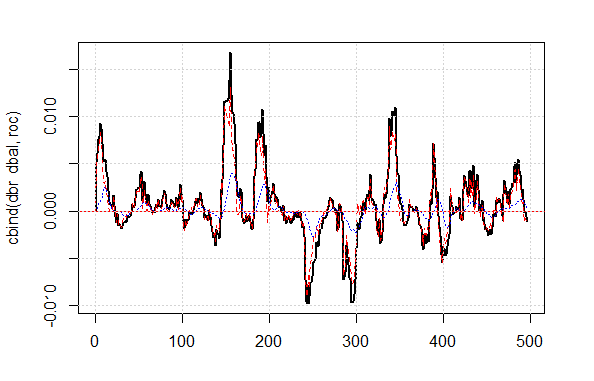```plot(c(NA,NA,NA,Bal), t="l")
lines(bal, col= 2)
lines(ma, col= 4)
```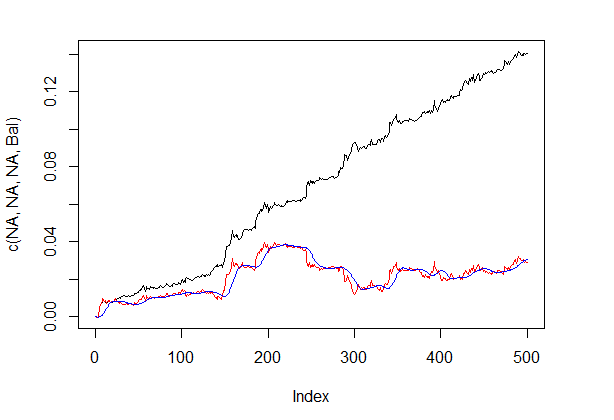```#---13----------------------------------
sig2stat <- function(x) {x %>% as.factor %>% as.numeric}
stat2sig <- function(x) ifelse(x==1, -1, 1)
#----14--调整-----------------------------------
correct <- function(sig){
sig <- Hmisc::Lag(sig) %>% na.omit
bal <- cumsum(sig * (price[ ,6] %>% tail(.,length(sig))))
ma <- pracma::movavg(bal, 16, "t")
roc <- TTR::momentum(ma, 3)%>% na.omit
dbal <- (bal - ma) %>% tail(., length(roc))
dbr <- (roc + dbal) %>% as.matrix()
sig.cor <- sign(dbr)
S <- sig.cor * tail(sig, length(sig.cor))
bal <- cumsum(S * (price[ ,6]%>% tail(.,length(S))))
K <- tail(bal, 1)/length(bal) * 10 ^ Dig
Kmax <- max(bal)/which.max(bal) * 10 ^ Dig
corr <<- list(sig.c = sig.cor, sig.res = S, bal = bal, Kmax = Kmax, K = K, dd = dd)
return(corr)
}
```

```#---15---马尔科夫链----------------------------------
pred.sig <- function(sig, prev.bar = 10, nahead = 1){
require(markovchain)
# 将观察到的校正信号转换为状态
stat <- sig2stat(sig)
# 计算模型参数
# 如果在环境中没有模型
if(!exists('MCbsp')){
MCbsp <<- markovchainFit(data = stat,
method = "bootstrap",
nboot = 10L,
name="Bootstrap MС")
}
# 设置必要常数
newData <- tail(stat, prev.bar)
pr <- predict(object = MCbsp\$estimate,
newdata = newData,
# 将预测信号挂载到输入信号
sig.pr <- c(sig, stat2sig(pr))
return(sig.pr = sig.pr)
}
```

```sig <- resBal[]\$sig
sig.cor <- correct(sig)
sig.c <- sig.cor\$sig.c
pr.sig.cor <- pred.sig(sig.c)
sig.pr <- pr.sig.cor\$sig.pr
# 用于智能交易系统的信号结果向量
S <- sig.pr * tail(sig, length(sig.pr))
```
• 平滑预测信号

```#---16---平滑------------------------------------
smoooth <- function(sig){
# 平滑预测信号
# 定义隐藏马尔科夫模型的参数
# 如果环境中尚无模型
require(mhsmm)
if(!exists('obj.sm')){
obj.sm <<- sig2stat(sig)%>% smooth.discrete()
}
# 用所获模型平滑信号
sig.s <- predict(obj.sm, x = sig2stat(sig))%>%
extract2(1)%>% stat2sig()
# 用平滑信号计算平衡
sig.s1 <- Hmisc::Lag(sig.s) %>% na.omit
bal <- cumsum(sig.s1 * (price[ ,6]%>% tail(.,length(sig.s1))))
K <- tail(bal, 1)/length(bal) * 10 ^ Dig
Kmax <- max(bal)/which.max(bal) * 10 ^ Dig
return(list(sig = sig.s, bal = bal, Kmax = Kmax, K = K, dd = dd))
}
```

```sig <- resBal[]\$sig
sig.sm <- smoooth(sig)
plot(sig.sm\$bal, t="l")
lines(resBal[]\$bal, col=2)
```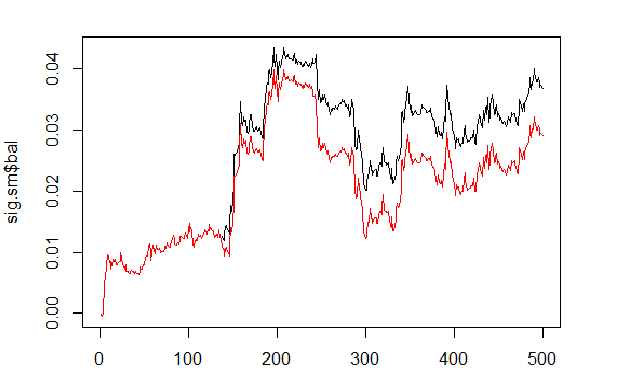```sig.sm\$dd
\$maxdrawdown
 0.02335
\$from
 208
\$to
 300
```

### 4. EA 算法的结构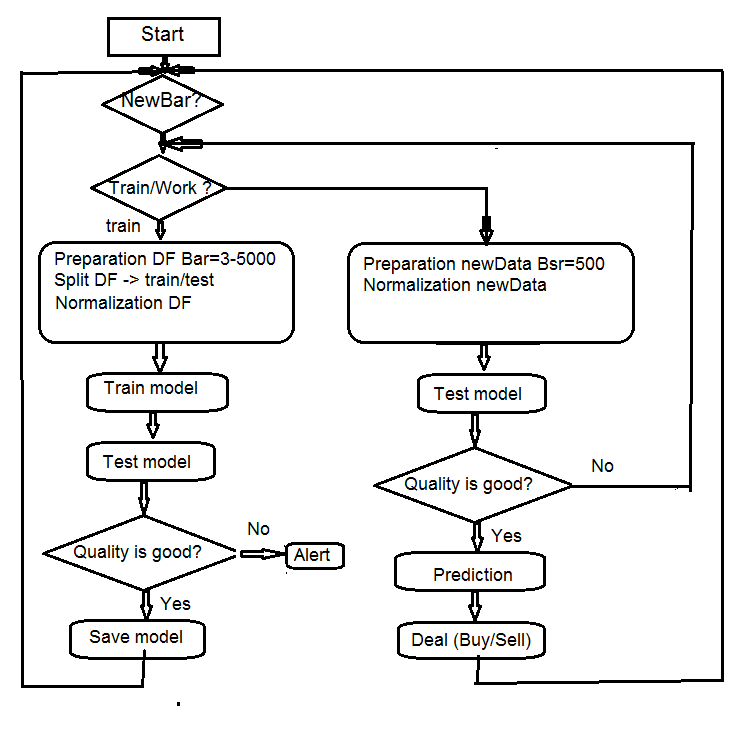### 4.1.1 MQL

init() 函数里

• 我们检查终端的设置 (DLL 可用, 授权交易);
• 设置定时器;
• 启动 Rterm;
• 计算并传递所需工作常量至 R-过程环境;
• 检查 Rterm 是否工作, 如果没有 - 报警;
• 从 init() 退出。

deinit() 函数里

• 我们停止定时器;
• 删除图形对象;
• 停止 Rterm。

onTimer()函数里

• 检查 Rterm 是否正在工作;
• 如果 Rterm 未占据且 新柱线 是 (LastTime != Time):
• 根据是否为首次启动 EA, 设置历史深度;
• 形成四个报价向量 (开盘价, 最高价, 最低价, 收盘价) 并将它们传递进 Rterm;
• 启动脚本并离开, 无需接收其执行结果;
• 设置 get_sig = true 标志;
• 设置 LastTime= Time。
• 否则, 若 Rterm 工作, 未占据且标志为 get_sig = true:
• 识别我们将从 Rterm 接收的 sig 向量的长度;
• 将接收向量的大小调整为源头的大小。当不可遵守时, Rprocess 将遗弃;
• 获取信号的顺序 (向量);
• 使用最后的信号来判定哪个操作已执行 (买入, 卖出, 无);
• 如果我们获得真实信号, 非 ERR, 我们会重置标志为 get_sig=false。
• 其余的为标准:
• CheckForClose()
• CheckForOpen()

### 4.1.2 Rterm

• 如果 首次:
• 从互联网上的存储库中加载 (若需要) 必要库文件, 并将它们安装到 Rterm 环境;
• 定义必要函数;
• 创建报价矩阵;
• 准备用于训练和测试模型的数据样本;
• 创建和训练模型;
• 测试模型;
• 计算执行信号;
• 检查预测品质。如果它高于或等于所设置的最小值 — 处理。否则 — 发送警报。
• 如果 非首次:
• 准备用于测试和预测的数据样本;
• 在新数据上测试模型;
• 计算执行信号;
• 检查预测品质。如果它超出或等于所设置的最小值 — 我们处理。否则 — 我们设置 first = TRUE, 即, 我们请求重新训练模型。

### 5. 安装和启动

• *.mq4 在 ~/MQL4/Expert 文件夹
• *.mqh 在 ~/MQL4/Include 文件夹
• *.dll 在 ~/MQL4/Libraries 文件夹
• *.r 在 C:/RData 文件夹

### 6. 改进定性指标的方式方法。

• 预测器的评估和选择 — 应用遗传算法优化 (GA)。
• 确定预测器和目标变量的最优参数 — GA。
• 确定神经网络的最优参数 — GA。

### 结论

• 深层神经网络, 初始 RBM 训练结果比 SAE 更差。这对我们来说并非新闻。
• 网络被快速训练。
• 通过对模型的几乎所有内部参数提供访问, 软件包具有改进预测品质的巨大潜力。
• 软件包仅可用在具有更广泛参数集合的神经网络或 RBM (相对其它标准的)。
• 软件包正在不断发展, 开发人员承诺在下一个版本中引入更多功能。
• R 语言与 МТ4/МТ5 终端的集成, 如开发者承诺, 将为交易者提供机会来使用最新的算法而无需额外的 DLL。

### 附件

1. R 语言的 Sess_DNRBM_GBPUSD_30 会话过程
2. "e_DNRBM" 智能交易系统的 ZIP 文件
3. "e_DNSAE" 智能交易系统的 ZIP 文件

DNSAE.zip (9.25 KB)
DNRBM.zip (112.57 KB)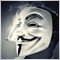| 3 2月 2017 在 01:49| 31 3月 2017 在 11:33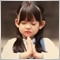| 30 9月 2018 在 06:11| 24 2月 2019 在 16:48

Обсуждение и вопросы по коду можно сделать в ветке

Удачи| 18 3月 2019 在 01:12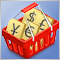在外汇市场中货币篮子的运作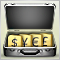在 MetaTrader 4 中的投资组合交易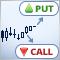如何构建和使用 MetaTrader 4的策略测试器来测试二元期权策略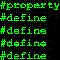在非标准时间范围上测试 Expert Advisor